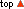music theory online : inversion of intervals lesson 13 Dr. Brian Blood to use the menu you must first enable javascript previous lesson :: next lesson :: contents :: index :: manuscript paper :: comments or queries? 1 | 2 | 3 | 4 | 5 | 6 | 7 | 8 | 9 | 10 | 11 | 12 | 13 | 14 | 15 | 16 | 17 | 18 | 19 | 20 | 21 | 22 | 23 | 24 | 25 | 26 | 27 | 28 | 29 | 30 | 31 | 32 | 33 | 34 | 35 | 36 | 37 | 38 | 39 | 40 | 41 | 42 | 43 | 44 But truth is so great a thing that we ought not to despise any medium that will conduct us to it.Michel Montaigne (1533-92) French essayist Inversion of Intervals

Inversion of Intervals ::Key word:
inversion
rule of 9
1

Inversion of Intervals

When discussing intervals, their degree (1st, 2nd, 3rd, etc.) and their quality (diminished, minor, major, etc.), we stressed the importance of working from the lower note and treating it like a key-note, so that all intervals are relative to the key-note. However, there will be occasions when particular dispositions of notes can be considered as inversions. For example, if two melodic lines are placed one above the other and the parts 'cross' so that some notes of the upper part (A) which generally lie above those of the lower part (B) now lie below those of part (B), we might want to discuss the intervals as being inverted. The chart below summarises the way this is done.we can summarise the general rules for 'renaming' intervals that have been inverted: 1 a 2nd becomes a 7th, a 3rd becomes a 6th, and so on 2 perfect intervals remain perfect 3 major intervals becomes minor intervals 4 minor intervals becomes major intervals 5 augmented intervals becomes diminished intervals 6 diminished intervals become augmented intervals 7 a tritone remains a tritone

 2 'Rule of 9' Inversion offers a neat way of working out the names of intervals larger than an augmented fourth. If you remember the inversional relationships in the table above, you only have to memorize the interval types to the augmented fourth. If you have an interval larger than an augmented fourth, simply invert it, identify the interval, then invert that. For example, the interval D-B flat is a sixth. Instead of counting semitones (half steps) to determine the type of sixth, invert it to B flat-D. Now you have a third, you can count the semitones (half steps), four, and determine that it is a major third. Therefore, your original interval is a minor sixth, the inversion of the major third For diatonically-named intervals, there is a useful rule applying to all simple intervals (that is those of one octave or less). The number of any interval and the number of its inversion always add up to nine. Thus a fifth (number 5) and its inverse or complement, a fourth (number is 4) add up to 9. Where intervals are identified by ratio, (for example, a fifth may be expressed as 3/2), the inversion is determined by reversing the ratio and multiplying by 2, in this case 4/3, which is the ratio of a fourth. Multiplying them together gives an octave 3/2 x 4/3 = 2/1.